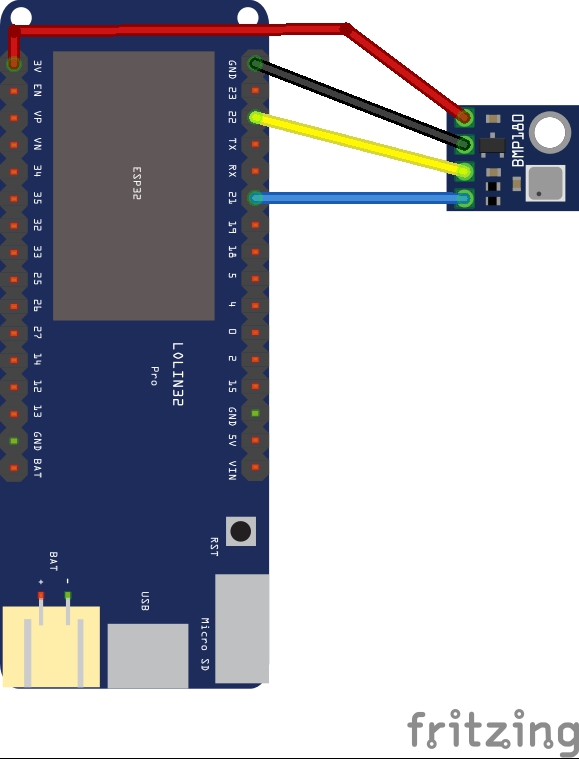Home Code A BMP180 Micropython example on an ESP32

# A BMP180 Micropython example on an ESP32

In this example we connect a BMP180 barometric pressure sensor to an ESP32 running Micropython

The BMP180 is the new digital barometric pressure sensor of Bosch Sensortec, with a very high performance, which enables applications in advanced mobile devices, such as smartphones, tablet PCs and sports devices. It follows the BMP085 and brings many improvements, like the smaller size and the expansion of digital interfaces.

The ultra-low power consumption down to 3 μA makes the BMP180 the leader in power saving for your mobile devices. BMP180 is also distinguished by its very stable behavior (performance) with regard to the independency of the supply voltage.

Applications

– GPS-enhancement for dead-reckoning, slope detection, etc.

– Sport devices, e.g. altitude profile

– Weather forecast

– Vertical velocity indication (rise/sink speed)

Parameter Technical data
Pressure range 300 … 1100 hPa
RMS noise expressed in pressure 0.06 hPa, typ. (ultra low power mode)
0.02 hPa, typ. (ultra high resolution mode)
RMS noise expressed in altitude 0.5 m, typ. (ultra low power mode)
0.17 m, typ. (ultra high resolution mode)
Relative accuracy pressure
VDD = 3.3 V
950 … 1050 hPa/ ±0.12 hPa
@ 25 °C/ ±1.0 m
700 … 900 hPa/ ±0.12 hPa
25 … 40 °C/ ±1.0 m
Absolute accuracy
p = 300…1100hPa
(Temperature = 0…+65°C, VDD = 3.3. V)
Pressure: -4.0 … +2.0 hPa
Temperature: ±1 °C, typ.
Average current consumption (1 Hz data refresh rate)

Peak current

3 μA, typical (ultra-low power mode)

650 μA, typical

Stand-by current 1.62 … 3.6 V
Supply voltage VDDIO 1.62 … 3.6 V
Supply voltage VDD 1.8 … 3.6 V
Operation temp.
Range full accuracy”
-40 … +85 °C
0 … +65 °C
Pressure conv. Time 5 msec, typical (standard mode)
I²C date transfer rate 3.4 MHz, max.

### Connection

An easy module to connect to an ESP32, SCL is 22 and SDA is 21 on the Wemos board I used, you can see this is the schematic belowesp32 and bmp180

### Code

You can use any method to upload files or an IDE for development, I used uPyCraft to u

The following is based on a github library – just search for Micropython bmp180. The first part of this is the library which I upload to my ESP32

This is the library called bmp180.py

[codesyntax lang=”python”]

```rom ustruct import unpack as unp
from machine import I2C, Pin
import math
import time

# BMP180 class
class BMP180():
'''
Module for the BMP180 pressure sensor.
'''

_bmp_addr = 119             # adress of BMP180 is hardcoded on the sensor

# init
def __init__(self, i2c_bus):

# create i2c obect
self._bmp_i2c = i2c_bus
self._bmp_i2c.start()
# read calibration data from EEPROM

# settings to be adjusted by user
self.oversample_setting = 3
self.baseline = 101325.0

# output raw
self.UT_raw = None
self.B5_raw = None
self.MSB_raw = None
self.LSB_raw = None
self.XLSB_raw = None
self.gauge = self.makegauge() # Generator instance
for _ in range(128):
next(self.gauge)
time.sleep_ms(1)

def compvaldump(self):
'''
Returns a list of all compensation values
'''
return [self._AC1, self._AC2, self._AC3, self._AC4, self._AC5, self._AC6,
self._B1, self._B2, self._MB, self._MC, self._MD, self.oversample_setting]

# gauge raw
def makegauge(self):
'''
Generator refreshing the raw measurments.
'''
delays = (5, 8, 14, 25)
while True:
t_start = time.ticks_ms()
while (time.ticks_ms() - t_start) <= 5: # 5mS delay
yield None
try:
except:
yield None
t_start = time.ticks_ms()
while (time.ticks_ms() - t_start) <= t_pressure_ready:
yield None
try:
except:
yield None
yield True

if next(self.gauge) is not None: # Discard old data
pass
while next(self.gauge) is None:
pass

@property
def oversample_sett(self):
return self.oversample_setting

@oversample_sett.setter
def oversample_sett(self, value):
if value in range(4):
self.oversample_setting = value
else:
print('oversample_sett can only be 0, 1, 2 or 3, using 3 instead')
self.oversample_setting = 3

@property
def temperature(self):
'''
Temperature in degree C.
'''
next(self.gauge)
try:
UT = unp('>H', self.UT_raw)
except:
return 0.0
X1 = (UT-self._AC6)*self._AC5/2**15
X2 = self._MC*2**11/(X1+self._MD)
self.B5_raw = X1+X2
return (((X1+X2)+8)/2**4)/10

@property
def pressure(self):
'''
Pressure in mbar.
'''
next(self.gauge)
self.temperature  # Populate self.B5_raw
try:
MSB = unp('B', self.MSB_raw)
LSB = unp('B', self.LSB_raw)
XLSB = unp('B', self.XLSB_raw)
except:
return 0.0
UP = ((MSB << 16)+(LSB << 8)+XLSB) >> (8-self.oversample_setting)
B6 = self.B5_raw-4000
X1 = (self._B2*(B6**2/2**12))/2**11
X2 = self._AC2*B6/2**11
X3 = X1+X2
B3 = ((int((self._AC1*4+X3)) << self.oversample_setting)+2)/4
X1 = self._AC3*B6/2**13
X2 = (self._B1*(B6**2/2**12))/2**16
X3 = ((X1+X2)+2)/2**2
B4 = abs(self._AC4)*(X3+32768)/2**15
B7 = (abs(UP)-B3) * (50000 >> self.oversample_setting)
if B7 < 0x80000000:
pressure = (B7*2)/B4
else:
pressure = (B7/B4)*2
X1 = (pressure/2**8)**2
X1 = (X1*3038)/2**16
X2 = (-7357*pressure)/2**16
return pressure+(X1+X2+3791)/2**4

@property
def altitude(self):
'''
Altitude in m.
'''
try:
p = -7990.0*math.log(self.pressure/self.baseline)
except:
p = 0.0
return p```

[/codesyntax]

Now you either update main.py or create it with the following code

[codesyntax lang=”python”]

```from bmp180 import BMP180
from machine import I2C, Pin                        # create an I2C bus object accordingly to the port you are using
#bus = I2C(1, baudrate=100000)           # on pyboard
bus =  I2C(scl=Pin(22), sda=Pin(21), freq=100000)   # on esp8266
bmp180 = BMP180(bus)
bmp180.oversample_sett = 2
bmp180.baseline = 101325

temp = bmp180.temperature
p = bmp180.pressure
altitude = bmp180.altitude
print("Temperature: ",temp)
print("Pressure: ",p)
print("Altitude: ",altitude)```

[/codesyntax]

### Testing

Open up the REPL window. Here is what I saw in uPyCraft